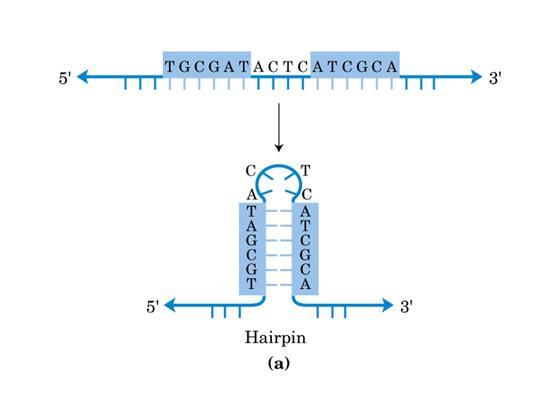# DNA zipperAs we know, DNA forms structured molecules through the attractive hydrogen bonding of guanine to cytosine, and of adenosine to thymine. Hairpins are single stranded molecules of DNA which fold back on themselves to form an open ended double helix with a loop at one end (see diagram above). Consider a very simple model of DNA hairpin binding where base $1$ can only bind with base $N$, base $2$ only with base $N-1$, and so on, so that altogether we can form a maximum of $N/2$ bonds. We can think of the allowable configurations as equivalent to those of a zipper.

We keep a dilute solution of thousands of these DNA molecules in a test tube. If each base-base bond has an enthalpy of $-35$ kJ/mol and an entropy of $-95$ J/mol/K$^\circ$, how many bonds (on average) do we expect to find in a hairpin of length $100$ bases (i.e. there can be at most 50 bonds) when the test tube is kept at $105$ C$^\circ$?

Hints

• Find the partition function of the DNA hairpin.
×